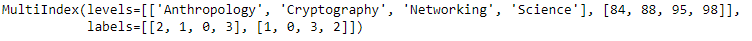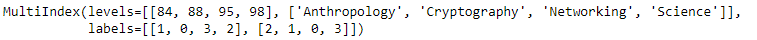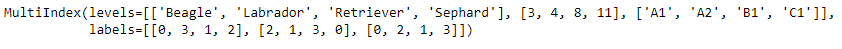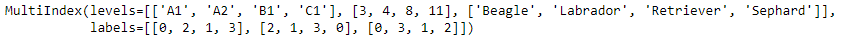Related Articles

# Python | Pandas MultiIndex.swaplevel()

• Last Updated : 24 Dec, 2018

Python is a great language for doing data analysis, primarily because of the fantastic ecosystem of data-centric python packages. Pandas is one of those packages and makes importing and analyzing data much easier.

Pandas` MultiIndex.swaplevel()` function is used to swap levels of the MultiIndex. It swap level i with level j. Calling this method does not change the ordering of the values.

Syntax: MultiIndex.swaplevel(i=-2, j=-1)

Parameters :
i : First level of index to be swapped. Can pass level name as string. Type of parameters can be mixed.
j : Second level of index to be swapped. Can pass level name as string. Type of parameters can be mixed.

Returns : A new MultiIndex

Example #1: Use `MultiIndex.swaplevel()` function to swap the 0th level with the 1st level of the MultiIndex.

 `# importing pandas as pd``import` `pandas as pd`` ` `# Create the MultiIndex``midx ``=` `pd.MultiIndex.from_arrays([[``'Networking'``, ``'Cryptography'``, ``                                     ``'Anthropology'``, ``'Science'``], ``                                             ``[``88``, ``84``, ``98``, ``95``]])`` ` `# Print the MultiIndex``print``(midx)`

Output :Now let’s swap the 0th level with the 1st level of the MultiIndex.

 `# swap the levels``midx.swaplevel(``0``, ``1``)`

Output :As we can see in the output, the function has swapped the 0th level with the 1st level of the MultiIndex.

Example #2: Use `MultiIndex.swaplevel()` function to swap the 0th level with the 1st level of the MultiIndex.

 `# importing pandas as pd``import` `pandas as pd`` ` `# Create the MultiIndex``midx ``=` `pd.MultiIndex.from_arrays([[``'Beagle'``, ``'Sephard'``, ``'Labrador'``, ``'Retriever'``],``                                       ``[``8``, ``4``, ``11``, ``3``], [``'A1'``, ``'B1'``, ``'A2'``, ``'C1'``]])`` ` `# Print the MultiIndex``print``(midx)`

Output :Now let’s swap the 0th level with the 2nd level of the MultiIndex.

 `# swap the levels``midx.swaplevel(``0``, ``2``)`

Output :As we can see in the output, the function has swapped the 0th level with the 2nd level of the MultiIndex.

Attention geek! Strengthen your foundations with the Python Programming Foundation Course and learn the basics.

To begin with, your interview preparations Enhance your Data Structures concepts with the Python DS Course. And to begin with your Machine Learning Journey, join the Machine Learning – Basic Level Course

My Personal Notes arrow_drop_up